# futoshiki

explanations:-

This is an easy futoshiki puzzle.

Object of the puzzle:-

Each row (I have numbered R1 to R5) and each column (A to E) must have the numbers 1,2,3,4 and 5.

The only help is the symbols :-more than and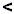less than

so, for example, the figure in R3C must be 1 or 2 as 3 is more than 1 or 2.

The four symbols given at the bottom link five cells so we know that the cells must be 5 down to 1

 A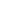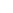BCDER1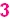R2R3R4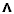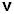R5